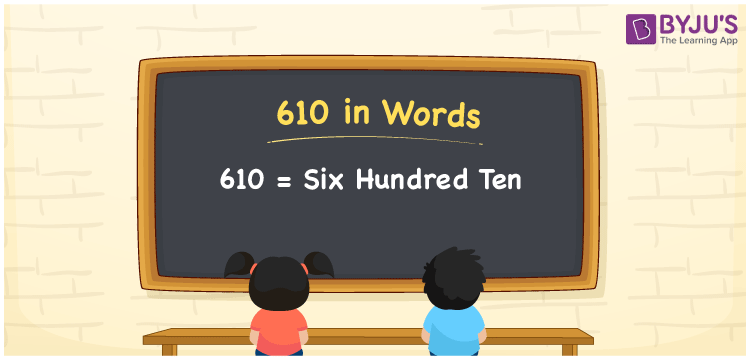# 610 in Words

610 in words can be written as Six Hundred Ten. The concepts like counting numbers which are important from primary education are discussed here in brief. For instance, if you spend Rs. 610 in buying curtains, then you can say that “I bought curtains for Six Hundred Ten Rupees”. The English alphabet is used to write 610 in words. Students can get a clear knowledge of numbers in words with the help of the study materials given at BYJU’S. The number 610 can be read as “Six Hundred Ten” in English.

 610 in words Six Hundred Ten Six Hundred Ten in Numbers 610

## 610 in English Words## How to Write 610 in Words?

Place value chart and expanded form of 610 can be learnt easily in this section. There are three digits in the number 610. The place value chart for 610 is provided below for reference to help students understand the concepts effectively.

 Hundreds Tens Ones 6 1 0

The expanded form of 610 is provided below:

6 × Hundred + 1 × Ten + 0 × One

= 6 × 100 + 1 × 10 + 0 × 1

= 610

= Six Hundred Ten

Hence, 610 in words is written as Six Hundred Ten.

610 is a natural number that precedes 611 and succeeds 609.

610 in words – Six Hundred Ten

Is 610 an odd number? – No

Is 610 an even number? – Yes

Is 610 a perfect square number? – No

Is 610 a perfect cube number? – No

Is 610 a prime number? – No

Is 610 a composite number? – Yes

## Frequently Asked Questions on 610 in Words

Q1

### How can 610 be written in words?

610 can be written as “Six Hundred Ten” in words.
Q2

### Is 610 an odd number?

No, 610 is an even number because it is divisible by 2.
Q3

### Write Six Hundred Ten in numbers.

Six Hundred Ten can be written in numbers as 610.